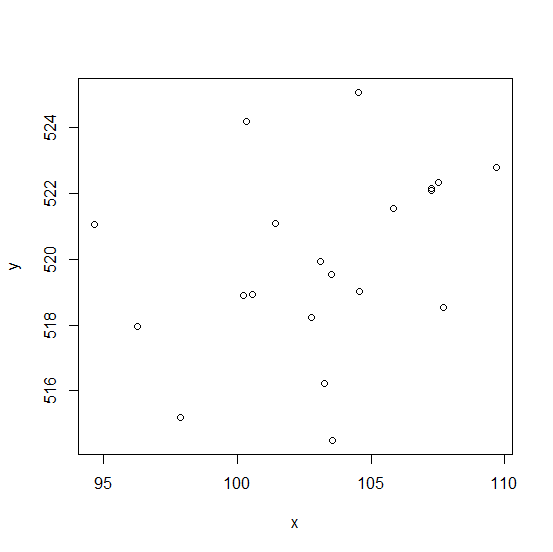# How to deal with the error ŌĆ£Error in int_abline---plot.new has not been called yetŌĆØ in R?

The above error means plot is not being created yet hence abline function cannot be used to draw anything on the plot. Therefore, a plot needs to be created first to use abline function for creating a line or any other thing. Mostly, abline is used to create regression line on the plot, thus we need to create a scatterplot first before using abline.

## Example

Live Demo

Consider the below data frame −

x<−rnorm(20,102,5.24)
y<−rnorm(20,520,3.27)
df<−data.frame(x,y)
df

## Output

x y
1 107.51245 522.3463
2 100.57137 518.9318
3 100.34198 524.1936
4 102.76870 518.2373
5 107.25869 522.0996
6 107.69508 518.5361
7 96.27562 517.9655
8 104.50759 525.0626
9 105.81623 521.5471
10 97.87036 515.1908
11 109.68729 522.7743
12 103.56346 514.5040
13 103.10188 519.9344
14 94.66500 521.0429
15 103.24753 516.2220
16 107.26232 522.1620
17 104.56150 519.0338
18 101.41522 521.0844
19 103.51826 519.5297
20 100.20963 518.9124

## Example

abline(lm(y~x))

## Output

Error in int_abline(a = a, b = b, h = h, v = v, untf = untf, ...) :
plot.new has not been called yet

To use abline, we first need to create the plot, hence it can be done as shown below −

## Example

plot(y~x,data=df)

## OutputCreating regression line using abline −

## Example

abline(lm(y~x))

## OutputUpdated on: 10-Feb-2021

3K+ Views